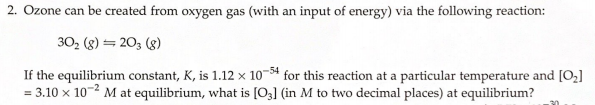# 2. Ozone can be created from oxygen gas (with an input of energy) via the following reaction: 302 (g) ⇌ 2O3 (g) If the equilibrium constant, K, is 1.12 x 10^-54 for this reaction at a particular temperature and [O2] = 3.10 x 10^-2 M at equilibrium, what is [O3] (in M to two decimal places) at equilibrium?